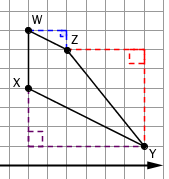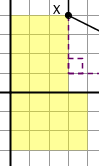### Home > GC > Chapter 6 > Lesson 6.2.2 > Problem6-52

6-52.

On graph paper, plot and connect the points to form quadrilateral $WXYZ$ if $W(3,7)$, $X(3,4)$, $Y(9,1)$, and $Z(5,6)$.

1. What is the shape of quadrilateral $WXYZ$? Justify your conclusion.

Quadrilateral $WXYZ$ looks like a trapezoid, but make sure by comparing the slopes of the sides that look parallel.

2. Find the perimeter of quadrilateral $WXYZ$.

Find the lengths of the sides that are not parallel to an axis by drawing slope triangles and using the Pythagorean Theorem.3. If quadrilateral $WXYZ$ is reflected using the transformation function ($x → −x$, $y→y$) to form quadrilateral $W'X'Y'Z'$ , then where is $Y'$?

Reflect the points across the $y$-axis by finding the distance between the point and axis and placing the reflected point that number of units to the other side of the axis.

Since $W$ is at $(3,7)$ and a $y$-axis reflection consists of $(x,y)$$(−x,y)$, then only the $x$-coordinate should change.
$W'$ should be plotted at $(−3,7)$.

Repeat this process for the other points!
Where should $X(3,4)$ be reflected to?

Plot it and name it $X'$.
Keep going with the other points.

4. Rotate quadrilateral $WXYZ$ about the origin $90°$ clockwise $(↻)$ to form quadrilateral $W''X''Y''Z''$. What is the slope of $\overleftrightarrow{W ^ { \prime \prime } Z ^ { \prime \prime }}$?

You may find it helpful to rotate points by drawing a rectangle between the origin and the point and then rotating the rectangle $90°$ clockwise.
Keep your eye on the point you're actually working on!See how the yellow rectangle has rotated $90°$ clockwise?

So $X''(4,-3)$

This slope can be written as $\frac{\Delta y}{\Delta x}$.

Use the eTool below to solve the parts of the problem.
Click the link at right for the full version of the eTool: 6-52 HW eTool (Desmos)